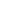# Free CBSE Class 7 Maths Data Handling Worksheets

Download free printable Data Handling Worksheets to practice. With thousands of questions available, you can generate as many Data Handling Worksheets as you want.

## Sample CBSE Class 7 Maths Data Handling Worksheet Questions

1.

Following data gives total marks (out of 700) obtained by 5 children. Represent the data by bar graphs.2.

Answer the following questions based on following graph:1).  In which subject did Seema obtained highest marks?

2).  In which subject did Seema obtained least marks?

3).  If 50 is the passing marks then name the subjects in which he got less than pass marks.

3.
Fill in the blanks: If a dice is thrown, the probability of getting a number less than 4 is ______.
4.
Fill in the blanks: If a dice is thrown, the probability of getting a prime number is ______.
5.
Fill in the blanks: If a dice is thrown, the probability of getting number 0 is ______.
6.
Fill in the blanks: For tossing a coin, the probability of getting tail is ______.
7.

Fill in the blanks: The probability of selecting the letter "A" from the word "ROAD" is _______.

8.
Fill in the blanks: The probability of an event always lies between _______ and 1.
9.
Fill in the blanks: The probability of an impossible event is _______.
10.
There are 6 marbles in a bag with number 1 to 6 marked on each of them. What is the probability of drawing a marble with number (1) 2, (2) 5?

## Find more Data Handling Worksheets

Worksheets by UrbanPro

Our worksheets are designed to help students explore various topics, practice skills and enrich their subject knowledge, to improve their academic performance. Designed by Experts who have extensive experience and expertise in teaching a subject, these worksheets will improve your child's problem-solving skills and subject knowledge in a fun and interactive manner.
Check out our free customized worksheets across school boards, grades, subjects and levels of subject knowledge. You can download, print and share these worksheets with anyone, anywhere, anytime!

Get a custom worksheet to practice!

Select your topic & see the magic.

subjectSelect Chapter(s)

Chapters & Subtopics